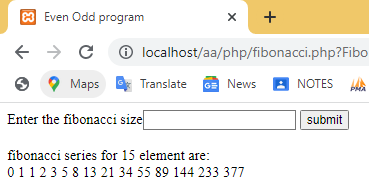# Fibonacci Series in PHP

The Fibonacci series starts with 0, and 1. a next number of the Fibonacci series is generated by adding the previous two numbers.

## Fibonacci series in php using while loop

``````<?php
\$a=0;
\$b=1;
\$num=0;
echo "fibonacci series for 10 element are:</br>";
echo \$a.'  '.\$b;
while(\$num<8)
{
\$next=\$a+\$b;
echo '  '.\$next;
\$a=\$b;
\$b=\$next;
\$num++;
}
?>``````

## output

```fibonacci series for 10 element are:
0  1  1  2  3  5  8  13  21  34```

## Program to print Fibonacci series in PHP by using for loop

``````<?php
\$a=0;
\$b=1;
echo "fibonacci series for 20 element are:</br>";
// print first two number
echo \$a.'  '.\$b;
/*since we have already printed first two number */
//Now we only iterate for loop from 1 to 18.
for(\$num=1;\$num<=18;\$num++)
{
// add previous two number and store in next variable
\$next=\$a+\$b;
echo '  '.\$next;
\$a=\$b;
\$b=\$next;
}
?>``````

## output

```fibonacci series for 20 element are:
0 1 1 2 3 5 8 13 21 34 55 89 144 233 377 610 987 1597 2584 4181
```

## Program to print Fibonacci series in php by using HTML form

In this example, you will take the length of series input from the user using HTML form and print the length of series by using a while loop.

``````<!DOCTYPE html>
<html lang="en">
<title>Program to print fibonaccie series</title>
<body>
<form>
Enter the fibonacci size<input type="number" name="Fibonacci">
<input type="submit" value="submit" name="submitForm"></br></br>
</form>
</body>
</html>
<?php
if(isset(\$_GET["submitForm"]))
{
\$num=\$_GET['Fibonacci'];
\$a=0;
\$b=1;
echo "fibonacci series for ".\$num." element are:</br>";
echo \$a.'  '.\$b;
\$i=0;
while(\$i<\$num-2)
{
\$next=\$a+\$b;
echo '  '.\$next;
\$a=\$b;
\$b=\$next;
\$i++;
}
}
?>``````

## output### Explanation of Fibonacci series program

a step-by-step explanation of the Fibonacci series program is given below:

1. Take Fibonacci series input from user in HTML form, after taking input from user click on submit button.
2. After submitting the form, the `\$_GET` method value will be set, and the `if-statement `evaluates the true expression.
3. Inside if-statement, you will store user input value in `\$num` variable by using code `\$num=\$_GET['Fibonacci'];`
4. Since the Fibonacci series starts with 0 or 1, therefore store 0 in` \$a` and 1 in `\$b`. Now print the value of \$a and \$b.
5. Since we have already printed the first two numbers i.e `\$a` and `\$b`, we iterate the while loop from `0` to less than `\$num - 2`.
6. Inside the while loop, add the previous two values and store the result in `\$next` variable by using code  `\$next=\$a+\$b`;
7. Print the `\$next` value and copy the value of` \$b` into `\$a` and `\$next` into `\$b`.
8. Increment the value of `i`. a loop will break when `i` value become `\$num-2`.